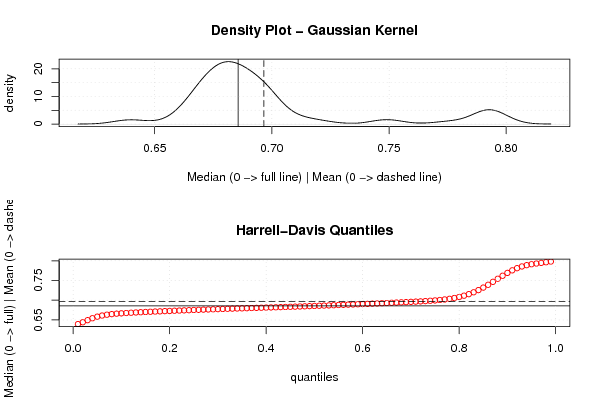## Free Statistics

of Irreproducible Research!

Author's title
Author*Unverified author*
R Software Modulerwasp_meanversusmedian.wasp
Title produced by softwareMean versus Median
Date of computationSun, 15 Mar 2009 10:50:59 -0600
Cite this page as followsStatistical Computations at FreeStatistics.org, Office for Research Development and Education, URL https://freestatistics.org/blog/index.php?v=date/2009/Mar/15/t123713589405ps3bggmn4kidr.htm/, Retrieved Thu, 30 Nov 2023 20:21:56 +0000
Statistical Computations at FreeStatistics.org, Office for Research Development and Education, URL https://freestatistics.org/blog/index.php?pk=39090, Retrieved Thu, 30 Nov 2023 20:21:56 +0000
QR Codes:Original text written by user:
IsPrivate?No (this computation is public)
User-defined keywordsWesley De Bondt
Estimated Impact193
Family? (F = Feedback message, R = changed R code, M = changed R Module, P = changed Parameters, D = changed Data)
-     [Histogram] [Koers Euro t.o.v....] [2009-03-15 14:07:45] [bc74728c7d756fc9a12b0f66e8f52b90]
- RMP     [Mean versus Median] [Koers Euro t.o.v....] [2009-03-15 16:50:59] [52abb83916effba29fe89a1e0cad1e5e] [Current]
Feedback Forum

Post a new message
Dataseries X:
0.63709
0.64218
0.65711
0.66977
0.68255
0.68902
0.71322
0.70224
0.70045
0.69919
0.69693
0.69763
0.69278
0.70196
0.69215
0.6769
0.67124
0.66533
0.67157
0.66428
0.66576
0.66942
0.68130
0.69144
0.69862
0.695
0.69867
0.68968
0.69233
0.68293
0.68399
0.66895
0.68756
0.68527
0.6776
0.68137
0.67933
0.67922
0.68598
0.68297
0.68935
0.69463
0.6833
0.68666
0.68782
0.67669
0.67511
0.67254
0.67397
0.67286
0.66341
0.668
0.68021
0.67934
0.68136
0.67562
0.6744
0.67766
0.68887
0.69614
0.70896
0.72064
0.74725
0.75094
0.77494
0.79487
0.79209
0.79152
0.79308
0.79279
0.79924
0.78668

 Summary of computational transaction Raw Input view raw input (R code) Raw Output view raw output of R engine Computing time 1 seconds R Server 'Gwilym Jenkins' @ 72.249.127.135

\begin{tabular}{lllllllll}
\hline
Summary of computational transaction \tabularnewline
Raw Input & view raw input (R code)  \tabularnewline
Raw Output & view raw output of R engine  \tabularnewline
Computing time & 1 seconds \tabularnewline
R Server & 'Gwilym Jenkins' @ 72.249.127.135 \tabularnewline
\hline
\end{tabular}
%Source: https://freestatistics.org/blog/index.php?pk=39090&T=0

[TABLE]
[ROW][C]Summary of computational transaction[/C][/ROW]
[ROW][C]Raw Input[/C][C]view raw input (R code) [/C][/ROW]
[ROW][C]Raw Output[/C][C]view raw output of R engine [/C][/ROW]
[ROW][C]Computing time[/C][C]1 seconds[/C][/ROW]
[ROW][C]R Server[/C][C]'Gwilym Jenkins' @ 72.249.127.135[/C][/ROW]
[/TABLE]
Source: https://freestatistics.org/blog/index.php?pk=39090&T=0

Globally Unique Identifier (entire table): ba.freestatistics.org/blog/index.php?pk=39090&T=0

As an alternative you can also use a QR Code:

The GUIDs for individual cells are displayed in the table below:

 Summary of computational transaction Raw Input view raw input (R code) Raw Output view raw output of R engine Computing time 1 seconds R Server 'Gwilym Jenkins' @ 72.249.127.135

 Median versus Mean mean 0.696554444444444 median 0.685625

\begin{tabular}{lllllllll}
\hline
Median versus Mean \tabularnewline
mean & 0.696554444444444 \tabularnewline
median & 0.685625 \tabularnewline
\hline
\end{tabular}
%Source: https://freestatistics.org/blog/index.php?pk=39090&T=1

[TABLE]
[ROW][C]Median versus Mean[/C][/ROW]
[ROW][C]mean[/C][C]0.696554444444444[/C][/ROW]
[ROW][C]median[/C][C]0.685625[/C][/ROW]
[/TABLE]
Source: https://freestatistics.org/blog/index.php?pk=39090&T=1

Globally Unique Identifier (entire table): ba.freestatistics.org/blog/index.php?pk=39090&T=1

As an alternative you can also use a QR Code:

The GUIDs for individual cells are displayed in the table below:

 Median versus Mean mean 0.696554444444444 median 0.685625PNG link Postscript link PDF link

Parameters (Session):
Parameters (R input):
R code (references can be found in the software module):
library(Hmisc)m <- mean(x)e <- median(x)bitmap(file='test1.png')op <- par(mfrow=c(2,1))mydensity1 <- density(x,kernel='gaussian',na.rm=TRUE)plot(mydensity1,main='Density Plot - Gaussian Kernel',xlab='Median (0 -> full line) | Mean (0 -> dashed line)',ylab='density')abline(v=e,lty=1)abline(v=m,lty=5)grid()myseq <- seq(0.01, 0.99, 0.01)hd <- hdquantile(x, probs = myseq, se = TRUE, na.rm = FALSE, names = TRUE, weights=FALSE)plot(myseq,hd,col=2,main='Harrell-Davis Quantiles',xlab='quantiles',ylab='Median (0 -> full) | Mean (0 -> dashed)')abline(h=m,lty=5)abline(h=e,lty=1)grid()par(op)dev.off()load(file='createtable')a<-table.start()a<-table.row.start(a)a<-table.element(a,'Median versus Mean',2,TRUE)a<-table.row.end(a)a<-table.row.start(a)a<-table.element(a,'mean',header=TRUE)a<-table.element(a,mean(x))a<-table.row.end(a)a<-table.row.start(a)a<-table.element(a,'median',header=TRUE)a<-table.element(a,median(x))a<-table.row.end(a)a<-table.end(a)table.save(a,file='mytable.tab')